Electromagnetic Induction

# Electromagnetic Induction - Basic Physics for IIT JAM

Electromagnetic Induction or Induction is a process in which a conductor is put in a particular position and magnetic field keeps varying or magnetic field is stationary and a conductor is moving. This produces a Voltage or  EMF (Electromotive Force) across the electrical conductor. Michael Faraday discovered Law of Induction in 1830. Let us now  study the  Electromagnetic Induction in detail.
Electromagnetic induction
Suppose while shopping you go cashless and  your parents use cards. The shopkeeper always scans or swipes the card. Shopkeeper does not take a photo of the card or tap it. Why does he swipe/scan it? And how does this swiping deduct money from the card? This happens because of the ‘Electromagnetic Induction’.
Can moving objects produce electric currents? How to determine a relationship between electricity and magnetism? Can you imagine the scenario if there were no computers, no telephones, no electric lights. The experiments of Faraday has led to the generation of generators and transformers.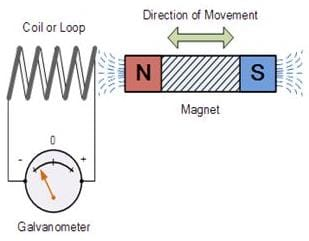The induction of an electromotive force by the motion of a conductor across a magnetic field or by a change in magnetic flux in a magnetic field is called ‘Electromagnetic Induction’.
This either happens when a conductor is set in a moving magnetic field (when utilizing AC power source) or when a conductor is always moving in a stationary magnetic field.
This law of electromagnetic induction was found by Michael Faraday. He organized a leading wire according to the setup given underneath, connected to a gadget to gauge the voltage over the circuit. So when a bar magnet passes through the snaking, the voltage is measured in the circuit. The importance of this is a way of producing electrical energy in a circuit by using magnetic fields and not just batteries anymore. The machines like generators,  transformers also the motors work on the principle of electromagnetic induction.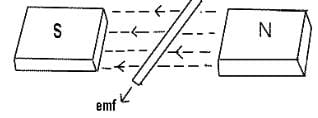• First law:  Whenever a conductor is placed in a varying magnetic field, EMF induces and this emf is called an induced emf and if the conductor is a closed circuit than the induced current flows through it.
• Second law: The magnitude of the induced EMF is equal to the rate of change of flux linkages.

Based on his experiments we now have Faraday’s law of electromagnetic induction according to which the amount of voltage induced in a coil is proportional to the number of turns and the changing magnetic field of the coil.
So now, the induced voltage is as follows: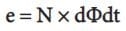where,
e is the induced voltage
N is the number of turns in the coil
Φ is the magnetic flux
t is the time

Lenz’s law of Electromagnetic Induction
Lenz law of electromagnetic induction states that, when an emf induces according to Faraday’s law, the polarity (direction) of that induced emf is such that it opposes the cause of its production.
According to Lenz’s law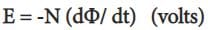Eddy currents
By Lenz law of electromagnetic induction, the current swirls in such a way as to create a magnetic field opposing the change. Because of the tendency of eddy currents to oppose, eddy currents cause a loss of energy. Eddy currents transform more useful forms of energy, such as kinetic energy, into heat, which isn’t generally useful. In many applications, the loss of useful energy is not particularly desirable, but there are some practical applications. Like:
1. In the brakes of some trains. During braking, the brakes expose the metal wheels to a magnetic field which generates eddy currents in the wheels. The magnetic interaction between the applied field and the eddy currents slows the wheels down. The faster the wheels spin, the stronger is the effect, meaning that as the train slows the braking force is reduces, producing a smooth stopping motion.
2. There are few galvanometers having a fixed core which are of nonmagnetic metallic material. When the coil oscillates, the eddy currents that generate in the core oppose the motion and bring the coil to rest.
3. Induction furnace can be used to prepare alloys, by melting the metals. The eddy currents generated in the metals produce high temperature enough to melt it.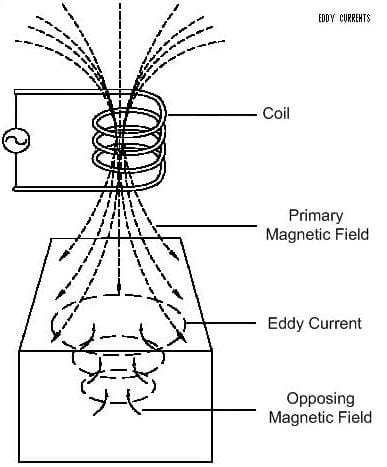Applications of Electromagnetic Induction
1. Electromagnetic induction in AC generator
2. Electrical Transformers
3. Magnetic Flow Meter
Electromagnetic induction in AC generator
One of the important application of electromagnetic induction is the generation of alternating current.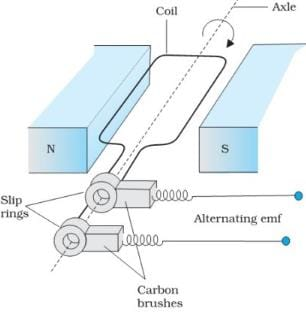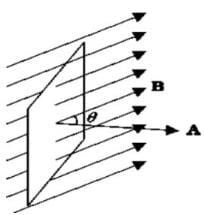The AC generator with an output capacity of 100 MV is a more evolved machine. As the coil rotates in a magnetic field B, the effective area of the loop is A cosθ, where θ is the angle between A and B. This is a method of producing a flux change is the principle of operation of a simple ac generator.  The axis of rotation coil is perpendicular to the direction of the magnetic field. The rotation of the coil causes the magnetic flux through it to change, so an emf keeps inducing in the coil.
Electrical Transformers
Another important application of electromagnetic induction is an electrical transformer. A transformer is a device that changes ac electric power at one voltage level to another level through the action of a magnetic field. A step-down transformer is the one in which the voltage is higher in the primary than the secondary voltage. Whereas the one in which the secondary voltage has more turns is a step-up transformer. Power companies use a step transformer to boost the voltage to 100 kV, that reduces the current and minimizes the loss of power in transmission lines. On the other end, household circuits use step-down transformers to decrease the voltage to the 120 or 240 V in them.

The document Electromagnetic Induction | Basic Physics for IIT JAM is a part of the Physics Course Basic Physics for IIT JAM.
All you need of Physics at this link: Physics

## FAQs on Electromagnetic Induction - Basic Physics for IIT JAM

 1. What is electromagnetic induction?Ans. Electromagnetic induction is the process of generating an electric current in a conductor by changing the magnetic field around it. According to Faraday's law of electromagnetic induction, whenever a conductor is exposed to a changing magnetic field, an electromotive force (EMF) is induced, which causes an electric current to flow in the conductor.
 2. How does electromagnetic induction work?Ans. Electromagnetic induction works based on the principle that a changing magnetic field induces an electric current in a conductor. When a conductor is moved through a magnetic field or when the magnetic field around the conductor changes, the flux linking the conductor changes, creating an EMF. This induced EMF then causes an electric current to flow through the conductor.
 3. What are the applications of electromagnetic induction?Ans. Electromagnetic induction has various practical applications. Some of the common applications include: - Electric generators: Electromagnetic induction is used to convert mechanical energy into electrical energy in generators. - Transformers: Transformers utilize electromagnetic induction to change the voltage of an alternating current. - Induction cooktops: These cooktops use electromagnetic induction to heat the cooking vessel directly. - Wireless charging: Electromagnetic induction is utilized in wireless charging technology to transfer power between devices without the need for physical connections. - Metal detectors: Metal detectors work based on electromagnetic induction, detecting changes in the magnetic field caused by metallic objects.
 4. What factors affect the magnitude of the induced EMF in electromagnetic induction?Ans. The magnitude of the induced EMF in electromagnetic induction depends on several factors, including: - The rate of change of the magnetic field: The faster the magnetic field changes, the greater the induced EMF. - The number of turns in the conductor: Increasing the number of turns in the conductor increases the induced EMF. - The strength of the magnetic field: A stronger magnetic field results in a higher induced EMF. - The angle between the magnetic field and the conductor: The induced EMF is maximum when the magnetic field is perpendicular to the conductor.
 5. Can electromagnetic induction be used to generate electricity from renewable energy sources?Ans. Yes, electromagnetic induction can be used to generate electricity from renewable energy sources. For example, wind turbines use the principle of electromagnetic induction to convert the kinetic energy of wind into electrical energy. The rotating blades of the wind turbine turn a generator, which contains coils of wire that experience a changing magnetic field as they rotate in the presence of a fixed magnetic field. This changing magnetic field induces an electric current in the coils, generating electricity. Similarly, hydroelectric power plants can also utilize electromagnetic induction to generate electricity by using flowing water to rotate a turbine connected to a generator.

## Basic Physics for IIT JAM

219 videos|156 docs|94 tests

## Basic Physics for IIT JAM

219 videos|156 docs|94 tests
Signup to see your scores go up within 7 days! Learn & Practice with 1000+ FREE Notes, Videos & Tests.
10M+ students study on EduRev
Track your progress, build streaks, highlight & save important lessons and more!(Scan QR code)
Related Searches

,

,

,

,

,

,

,

,

,

,

,

,

,

,

,

,

,

,

,

,

,

;